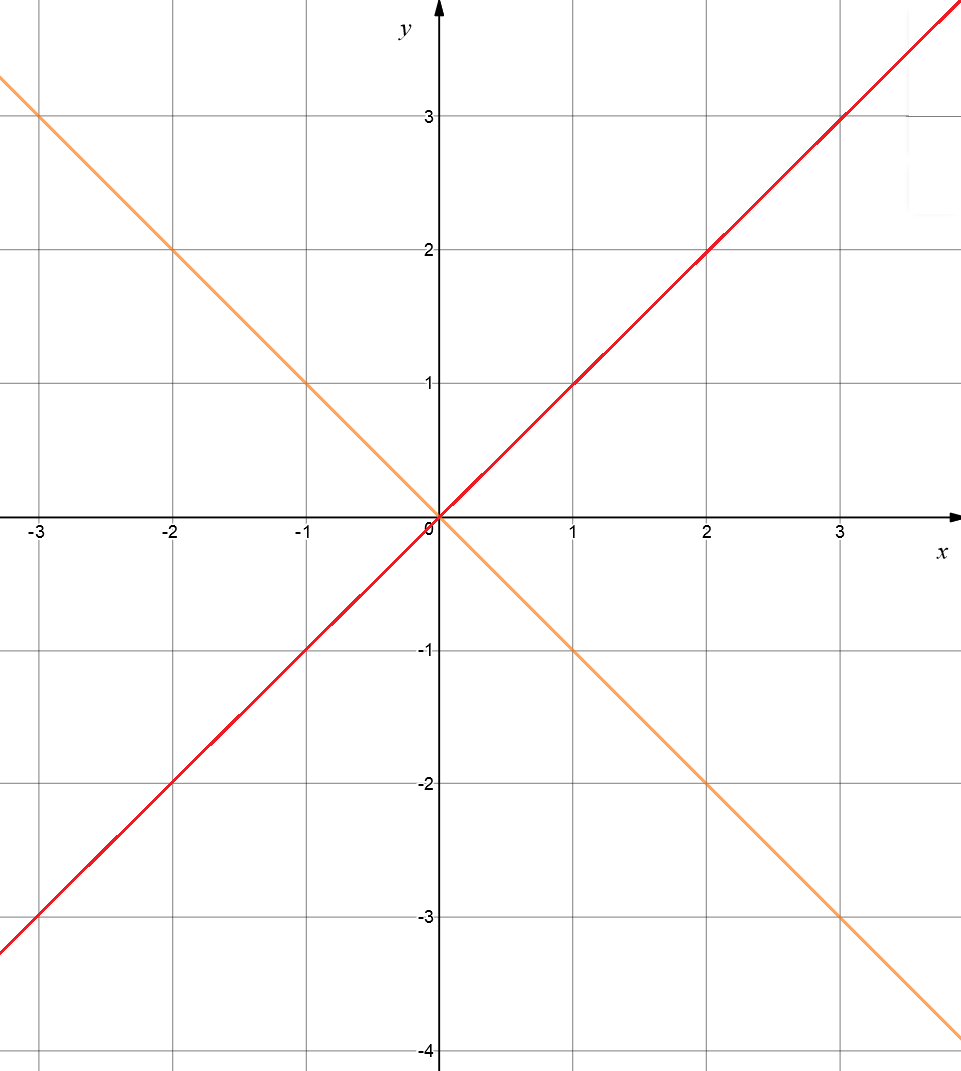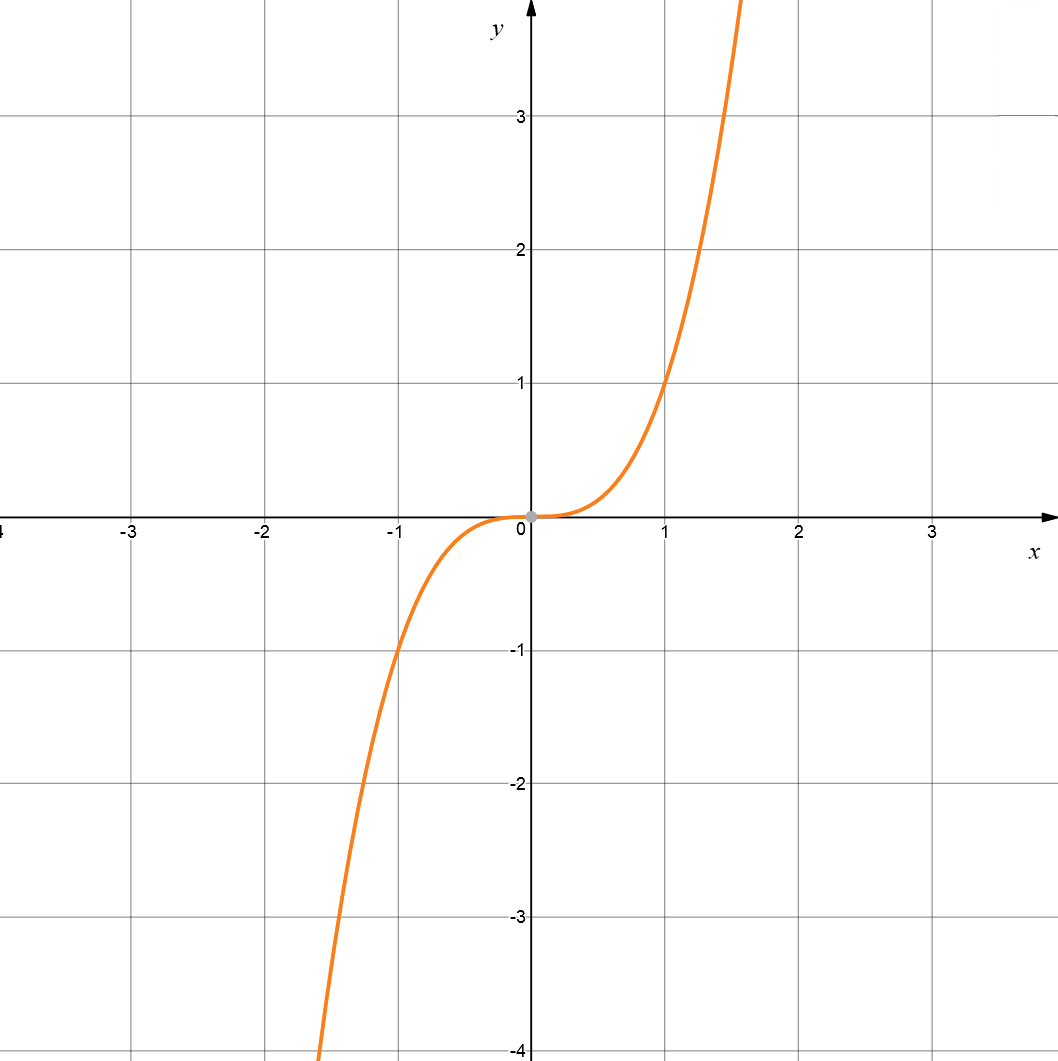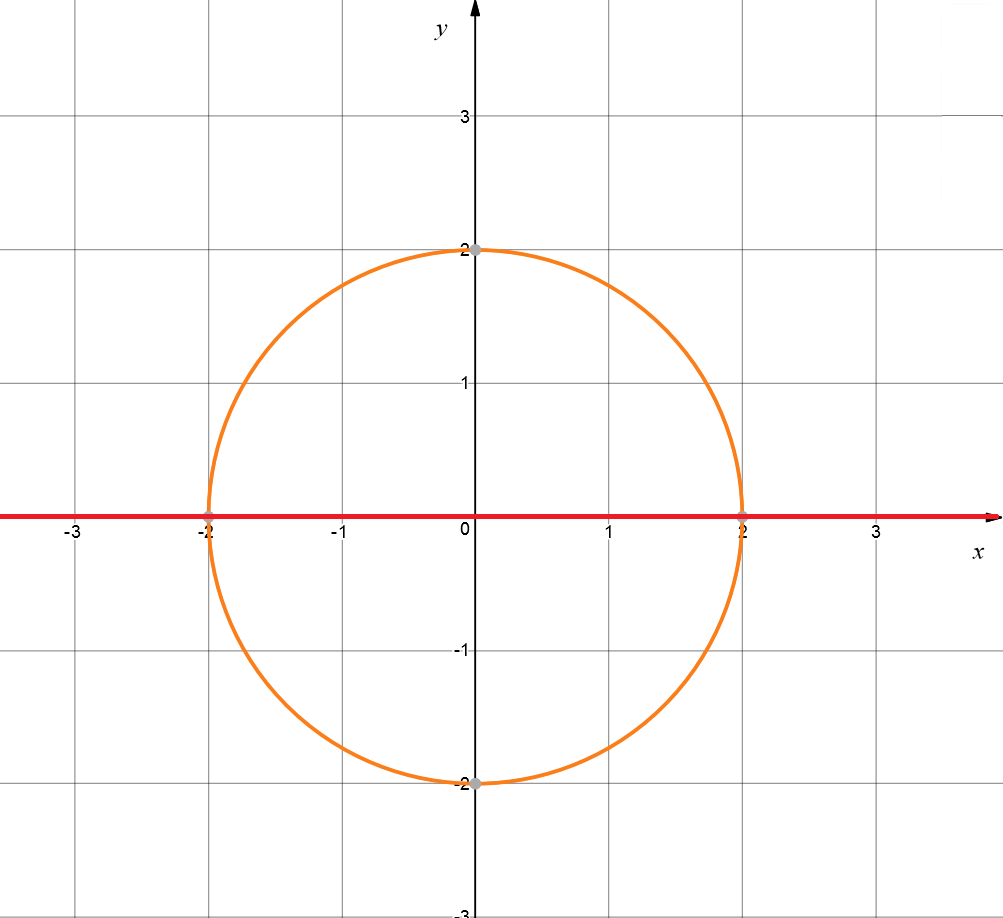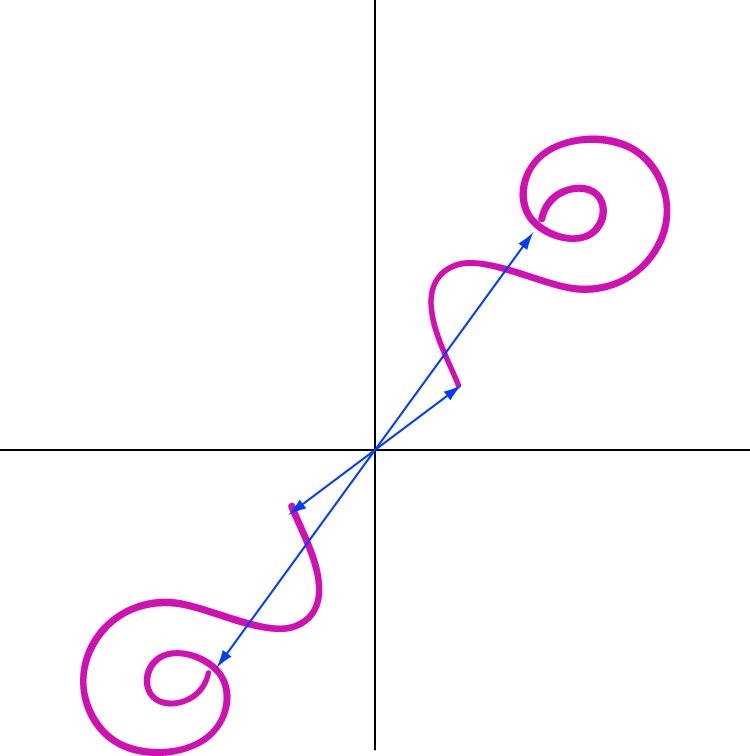Pull Down & Release to Refresh

# Symmetry in Equations

Equations can have many different types of symmetries. If an equation has symmetry, then its graph will have symmetry too!

Let's have a look at some examples of equations with symmetry.The graph of $y = x^4$

This has symmetry about the $y$-axis.The graph of $y = -x$

This has diagonal symmetry.The graph of $y = x^3$

This has point symmetry.

The red line marked in the first two graphs above is used to indicate the axis of symmetry of the graph.

## Advantages of Symmetry in Equations

• It can make the graph easier to plot: you only have to do half the work.
• It increase our understanding of the equation.
• We can use it to help us solve equations: if you know the equation is symmetric and you know one of the roots, you know that there's a corresponding root in the mirror image.

## Checking for Symmetry

We can use algebra! If we can find symmetric values, for example $x$ and $-x$, at which the equation gives the same result, then the equation is symmetric.

The sort of symmetric values we use depend on the type of symmetry that we're looking for.

### Symmetry about the $y$-axis

To check for symmetry about the $y$-axis, we check to see if the equation gives us the same value at $x$ and $-x$. Of course, this has to work for every allowed value of $x$.

#### Example

Is $y = x^4$ symmetric about the $y$-axis?

Solution:

Try putting a $-x$ wherever you see $x$ in the equation and see what happens:

\begin{align*} y &= (-x)^4\\ &= x^4 \end{align*}
as taking an even power of a negative number gives you a positive number. So, there's no change to the value of the equation.

$y = x^4$ is symmetric about the $x$-axis.

### Symmetry about the $x$-axis

This time, to check for symmetry about the $x$-axis, we check to see if the equation changes if we replace $y$ by $-y$.

#### Example

Is $x^2 + y^2 = 4$ symmetric about the $x$-axis?Solution:

Try putting $-y$ wherever you see $y$ in the equation:

\begin{align*} x^2 + (-y)^2 &= 4\\ x^2 + y^2 &= 4 \end{align*}
as taking the square of a negative number gives you a positive number. So, there's no change to the equation.

$x^2 + y^2 = 4$ is symmetric about the $x$-axis.

### Diagonal Symmetry

To test for diagonal symmetry, we swap the roles of $y$ and $x$ in the equation and see whether it changes. If it doesn't, the equation has diagonal symmetry.

#### Example

Does $y = x^3$ have diagonal symmetry?

Solution:

If we swap the roles of $x$ and $y$, then the equation becomes

$x = y^3$,
which is quite different from the original equation. So, $y = x^3$ does not have diagonal symmetry.

However, $y = -x$ does have diagonal symmetry. Swapping the roles of $y$ and $x$ yields

\begin{align*} x &= -y\\ -x &= y \mbox{ after multiplying both sides by } -1. \end{align*}

### Point SymmetryPoint symmetry is the same as rotational symmetry of order $2$. It occurs when every point of the graph has a matching point that is

• The same distance from the origin
• In the opposite direction

To test for point symmetry, we check to see whether we get the same equation after replacing $x$ by $-x$, and $y$ by $-y$.

#### Example

Does the equation $y = x^3$ have point symmetry?

Solution:

Try replacing $x$ with $-x$ and $y$ with $-y$. The equation becomes:

\begin{align*} -y &= (-x)^3\\ -y &= -x^3\\ y &= x^3 \mbox{ after multiplying both sides by -1.} \end{align*}
This is the original equation, so $y = x^3$ has point symmetry.

### Conclusion

Now it's your turn. Test some equations for symmetry. Perhaps you could start with $y = x^2$ and $y = \dfrac{1}{x}$. What type of symmetry do they have? I'll give you a hint: one of them has symmetry about the $y$-axis, and the other has both diagonal and point symmetry. Which is which? See if you can use algebra to check.

### Description

coordinate geometry is a branch of geometry where the position of the points on the plane is defined with the help of an ordered pair of numbers also known as coordinates. In this tutorial series, you will learn about vast range of topics such as Cartesian Coordinates, Midpoint of a Line Segment etc

### Audience

year 10 or higher, several chapters suitable for Year 8+ students.

### Learning Objectives

Explore topics related to Coordinates Geometry

Author: Subject Coach
You must be logged in as Student to ask a Question.